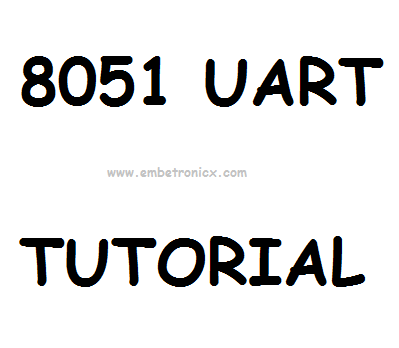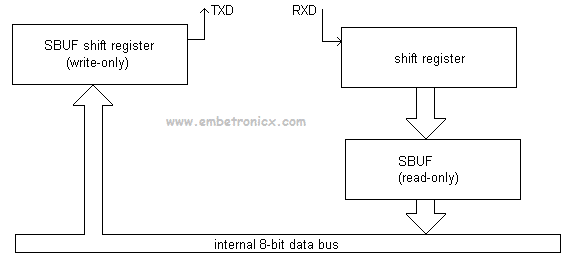# 8051 – UART Tutorial

Hi guys today we are going to see how to transfer data using UART and how to use UART’s SFR (8051 UART Tutorial). Before that you should know about the basic Serial Communication. Please see Here then you can understand this.

Now we can go to our topic.

# Introduction

The microcontroller MCS51 has an inbuilt UART for carrying out serial communication. The serial communication is done in the asynchronous mode. A serial port, like other PC ports, is a physical interface to establish data transfer between computer and an external hardware or device. This transfer, through serial port, takes place bit by bit.

Bit Addressable : We can assign the values bit by bit. For example for single bit we can set weather 1 or 0.

Byte Addressable : We cant assign the values bit by bit. We can set only byte by byte.

First we will see the SFRs.

# Registers used for UART

• SCON (Serial Control Register)     – Bit Addressable
• SBUF (Serial Buffer Register)        – Byte Addressable
• PCON (Power Control Register)    – Byte Addressable

## SCON (Serial Control Register)

This register is bit addressable.

 SM0 SM1 SM2 REN TB8 RB8 TI RI

SM0 : Serial Port Mode Specifier 1 bit.

SM1 : Serial port Mode Specifier 2 bit

These Two bits are used to select the Mode of the UART.

 SM0 SM1 Mode Baudrate 0 0 Shift Register (Mode 0) Fosc/12 0 1 8-bit UART (Mode 1) Variable (Set by Timer 1) 1 0 9-bit UART (Mode 2) Fosc/32 or Fosc/64 1 1 9-bit UART (Mode 3) Variable (Set by Timer 1)

SM2 : Multiprocessor communications bit. Set/cleared by program to enable multiprocessor communications in modes 2 and 3. When set to 1 an interrupt is generated if bit 9 of the received data is a 1; no interrupt is generated if bit 9 is a 0. If set to 1 for mode 1, no interrupt will be generated unless a valid stop bit is received. Clear to 0 if mode 0 is in use.

REN : Receive enable bit. Set to 1 to enable reception; cleared to 0 to disable reception.

TB8 :  Transmitted bit 8. Set/cleared by program in modes 2 and 3.

RB8 : Received bit 8. Bit 8 of received data in modes 2 and 3; stop bit in mode1. Not used in mode 0.

TI     : Transmit Interrupt flag. Set to one at the end of bit 7 time in mode 0, and at the beginning of the stop bit for other modes. Must be cleared by the program.

RI     :  Receive Interrupt flag. Set to one at the end of bit 7 time in mode 0, and halfway through the stop bit for other moves. Must be cleared by the program.

### Explanation

The first four bits (bits 4 through 7) are configuration bits. Bits SM0 and SM1 let us set the serial mode to a value between 0 and 3, inclusive. The four modes are defined in the chart immediately above. As you can see, selecting the Serial Mode selects the mode of operation (8-bit/9-bit, UART or Shift Register) and also determines how the baud rate will be calculated. In modes 0 and 2 the baud rate is fixed based on the oscillator’s frequency. In modes 1 and 3 the baud rate is variable based on how often Timer 1 overflows. We’ll talk more about the various Serial Modes in a moment.

The next bit, SM2, is a flag for “Multiprocessor communication.” Generally, whenever a byte has been received the 8051 will set the “RI” (Receive Interrupt) flag. This lets the program know that a byte has been received and that it needs to be processed. However, when SM2 is set the “RI” flag will only be triggered if the 9th bit received was a “1”. That is to say, if SM2 is set and a byte is received whose 9th bit is clear, the RI flag will never be set. This can be useful in certain advanced serial applications. For now it is safe to say that you will almost always want to clear this bit so that the flag is set upon reception of any character.

The next bit, REN, is “Receiver Enable.” This bit is very straightforward: If you want to receive data via the serial port, set this bit. You will almost always want to set this bit.

The last four bits (bits 0 through 3) are operational bits. They are used when actually sending and receiving data. They are not used to configure the serial port.

The TB8 bit is used in modes 2 and 3. In modes 2 and 3, a total of nine data bits are transmitted. The first 8 data bits are the 8 bits of the main value, and the ninth bit is taken from TB8. If TB8 is set and a value is written to the serial port, the data’s bits will be written to the serial line followed by a “set” ninth bit. If TB8 is clear the ninth bit will be “clear.”

The RB8 also operates in modes 2 and 3 and functions essentially the same way as TB8, but on the reception side. When a byte is received in modes 2 or 3, a total of nine bits are received. In this case, the first eight bits received are the data of the serial byte received and the value of the ninth bit received will be placed in RB8.

TI means “Transmit Interrupt.” When a program writes a value to the serial port, a certain amount of time will pass before the individual bits of the byte are “clocked out” the serial port. If the program were to write another byte to the serial port before the first byte was completely output, the data being sent would be garbled. Thus, the 8051 lets the program know that it has “clocked out” the last byte by setting the TI bit. When the TI bit is set, the program may assume that the serial port is “free” and ready to send the next byte.

Finally, the RI bit means “Receive Interrupt.” It functions similarly to the “TI” bit, but it indicates that a byte has been received. That is to say, whenever the 8051 has received a complete byte it will trigger the RI bit to let the program know that it needs to read the value quickly, before another byte is read.

## SBUF (Serial Buffer Register)

1. SBUF Register: For a byte of data to be transferred via the TxD line, it must be placed in the SBUF.
2. SBUF holds the byte of data when it is received by the MCS51’s RxD line.## PCON (Power Control Register)

This Register is not Bit Addressable.

 SMOD – – – GF1 GF0 PD IDL

SMOD : Double baud rate bit. If Timer 1 is used to generate baud rate and SMOD = 1, the baud rate is doubled when the serial port is used in modes 1, 2, or 3.

GF1      : General-purpose flag bit.

GF0     :  General-purpose flag bit.

PD        : Power Down bit. Setting this bit activates the Power Down operation in the 8051BH. (Available only in CHMOS).

IDL      : Idle Mode bit. Setting this bit activates Idle Mode operation in the 8051BH. (Available only in CHMOS).

# Initialize the UART (Configuration)

That’s all about Registers. When using the integrated serial port, obviously configure it. This lets us tell the 8051 how many data bits we want, the baud rate we will be using, and how the baud rate will be determined.

Here we are going to use Mode 1. Because that is 8-bit UART and we can generate Baudrate using Timer 1. If you dont know about the timer please click Here for the reference.

So Mode 1 means we have to give 0x50 value to the SCON Register.

## Generating Baudrate using Timer 1

Once the Serial Port Mode has been configured, as explained above, the program must configure the serial port’s baud rate. This only applies to Serial Port modes 1 and 3.

The Baud Rate is determined based on the oscillator’s frequency when in mode 0 and 2. In mode 0, the baud rate is always the oscillator frequency divided by 12. This means if you’re crystal is 11.0592Mhz, mode 0 baud rate will always be 921,583 baud. In mode 2 the baud rate is always the oscillator frequency divided by 64, so a 11.059Mhz crystal speed will yield a baud rate of 172,797.

In modes 1 and 3, the baud rate is determined by how frequently timer 1 overflows. The more frequently timer 1 overflows, the higher the baud rate. There are many ways one can cause timer 1 to overflow at a rate that determines a baud rate, but the most common method is to put timer 1 in 8-bit auto-reload mode (timer mode 2) and set a reload value (TH1) that causes Timer 1 to overflow at a frequency appropriate to generate a baud rate.

To determine the value that must be placed in TH1 to generate a given baud rate, we may use the following equation (assuming PCON.7 is clear).

### Calculation

`TH1 = 256 - ((Crystal / 384) / Baud)`

If PCON.7 is set then the baud rate is effectively doubled, thus the equation becomes:

`TH1 = 256 - ((Crystal / 192) / Baud)`

For example, if we have an 11.0592Mhz crystal and we want to configure the serial port to 19,200 baud we try plugging it in the first equation:

`TH1 = 256 - ((Crystal / 384) / Baud)`
` TH1 = 256 - ((11059000 / 384) / 19200 )`
` TH1 = 256 - ((28,799) / 19200)`
` TH1 = 256 - 1.5 = 254.5`

As you can see, to obtain 19,200 baud on a 11.059Mhz crystal we’d have to set TH1 to 254.5. If we set it to 254 we will have achieved 14,400 baud and if we set it to 255 we will have achieved 28,800 baud. Thus we’re stuck…

But not quite… to achieve 19,200 baud we simply need to set PCON.7 (SMOD). When we do this we double the baud rate and utilize the second equation mentioned above. Thus we have:

`TH1 = 256 - ((Crystal / 192) / Baud)`
` TH1 = 256 - ((11059000 / 192) / 19200)`
` TH1 = 256 - ((57699) / 19200)`
` TH1 = 256 - 3 = 253`

Here we are able to calculate a nice, even TH1 value. Therefore, to obtain 19,200 baud with an 11.059MHz crystal we must:

1. Configure Serial Port mode 1 or 3.
2. Configure Timer 1 to timer mode 2 (8-bit auto-reload).
3. Set TH1 to 253 to reflect the correct frequency for 19,200 baud.
4. Set PCON.7 (SMOD) to double the baud rate.

# Programming For UART

## Program 1

This program is used to send the data “embetronicx” via serial port to the computer.

## Output 1## Program 2

In this program i have added the receiver code also. This code sends the data to 8051 microcontroller whatever i’m typing in the keyboard of the computer. Then microcontroller again resend the data to computer.

## Output 2That’s all guys… Hope you have understood. If you have any doubt please ask us by commenting below. So i will give you the task.